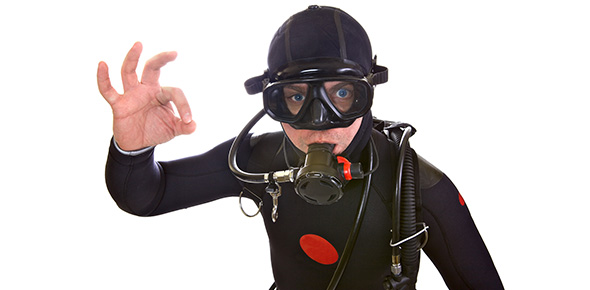# Chapter 5: Diving Physics

27 Questions | Total Attempts: 678SettingsSSI Divecon course dead scientist chapter

• 1.
Water is denser than air.
• A.

True

• B.

False

• 2.
A cubic foot of salt water weighs ___ pounds
• 3.
A cubic foot of fresh water weighs ___ pounds
• 4.
One atmosphere is ___ feet.
• 5.
____ is the relative hotness or coldness of a body or environment as measured on a standard scale, while ____ is defined as a form of energy that can be transmitted through solid or fluid medium by donduction, through  fluid medium by convection, or through a space as infrared radiation.
• A.

Heat / temperature

• B.

Cold / sound

• C.

Temperature / heat

• D.

Energy / light

• 6.
Water absorbs heat ____ times faster than air.
• 7.
The "pulling away" of heat in cold water is a property of heat energy transmission known as:
• A.

Conduction

• B.

Convection

• C.

• D.

Microwave

• 8.
Atmospheric pressure at One Atomsophere = _____ PSI.
• 9.
The sum of atmospheric pressure and hydrostatic pressure is:
• A.

Gauge pressure

• B.

Absolute pressure

• C.

Sum pressure

• D.

Under pressure

• E.

Ambient pressure

• 10.
The pressur surrounding you, whether you are underwater or above water is:
• A.

Absolute pressure

• B.

Gauge pressure

• C.

Under pressure

• D.

Partial pressure

• E.

Ambient pressure

• 11.
At a given pressure, the pressure of a single gas within a mixture of gases is.
• A.

Ambient pressure

• B.

Atmospheric pressure

• C.

Partial pressure

• D.

Absolute pressure

• E.

Gauge pressure

• 12.
The total pressure exerted by a mixture of gases is the sum of the pressures that would be exerted by each of the gases if it alone were present and occupied the total volume.
• A.

Archimedes' Principle

• B.

Dalton's Law

• C.

Boyle's Law

• D.

Henry's Law

• E.

Charles' Law

• 13.
What is the partial pressure of nitrogen at sea level? ____ PSI
• 14.
For any gas at a constant temperature, the volume will vary inversely with the absolute pressure while the density will vary directly with the absolute pressure.  This is:
• A.

Dalton's Law

• B.

Boyle's Law

• C.

Archimedes' Principle

• D.

Charles' Law

• E.

Henry's Law

• 15.
When pressure increases, volume of the gas ______.  When pressure decreases, volume of a gas ______.
• A.

Decreases / increases

• B.

Increases / decreases

• 16.
A gas is using 120 cubic feet at the surface.  What volume will it be at 66 feet? ____ cubic feet
• 17.
For any gas at a constant pressure, the volume of the gas will vary directly with the absolute temperature.  For any gas at a constant volume, the presure of the gas will vary directly with the absolute temperature.  This is:
• A.

Henry's Law

• B.

Archimedes' Principle

• C.

Boyle's Law

• D.

Charles' Law

• E.

Dalton's Law

• 18.
The amount of any given gas that will dissolve in a liquid at a given temperature is a function of the partial pressure of that gas in contact with the liquid and the solubility coefficient of the gas in the particular liquid.  This is:
• A.

Charles' Law

• B.

Boyle's Law

• C.

Dalton's Law

• D.

Henry's Law

• E.

Archimede's Principle

• 19.
A body immersed in liquid, either wholly or partially, is buoyed up by a force equal to the weight of the liquid displaced by the body.  This is:
• A.

Archimede's Principle

• B.

Boyle's Law

• C.

Charles' Law

• D.

Dalton's Law

• E.

Henry's Law

• 20.
You will be less buoyant in salt water than fresh.
• A.

True

• B.

False

• 21.
_____, which is the bending of light waves, results when light waves pass through mediums of different densities, and changing speed.
• 22.
Objects appear ___% closer underwater.
• 23.
Objects appear ___% larger underwater.
• 24.
What color disappears first underater?
• A.

Yellow

• B.

Green

• C.

Red

• D.

Orange

• 25.
Sound travels ___ times faters through water than air.
Related Topics